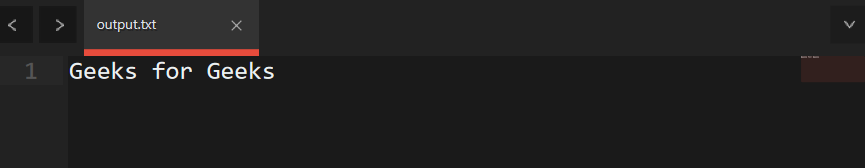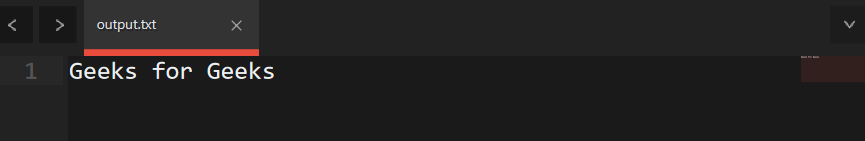Open in App
Not now

# How to iterate over the elements of an std::tuple in C++

• Difficulty Level : Medium
• Last Updated : 20 May, 2021

A C++ tuple is a container that can store multiple values of multiple types in it. We can access the elements of the tuple using std::get(), but std::get() always takes a constant variable parameter, so we can not simply iterate through it using a loop. For tasks that require iterating through all elements of the tuple. like printing all elements.

Below is the program to illustrate the iterating over an element tuple:

## CPP14

 `// C++ program to iterate over the``// elements of an std::tuple``// using std:get()``#include ``#include ``#include ` `// Driver Code``int` `main()``{``    ``// Declare a tuple and initialize``    ``// it using its constructor``    ``std::tuple``        ``tup(``"Geeks"``, ``"for"``, ``"Geeks"``);``    ``std::cout << ``"Values of tuple: "``;` `    ``// std::get is used to access``    ``// the value of tuple.``    ``std::cout << std::get<0>(tup)``              ``<< ``" "` `<< std::get<1>(tup)``              ``<< ``" "` `<< std::get<2>(tup)``              ``<< std::endl;` `    ``// Make the tuple using``    ``// std::make_tuple function``    ``tup = std::make_tuple(``"Hey"``, ``"Welcome to"``,``                          ``"Geeksforgeeks"``);` `    ``// Print tuple``    ``std::cout << ``"Values of tuple(Modified): "``;``    ``std::cout << std::get<0>(tup) << ``" "``              ``<< std::get<1>(tup) << ``" "``              ``<< std::get<2>(tup)``              ``<< std::endl;` `    ``return` `0;``}`

Output:

```Values of tuple: Geeks for Geeks
Values of tuple(Modified): Hey Welcome to Geeksforgeeks```

The problem arises when we try to iterate through the whole tuple. So, we have two methods here, to iterate through the values of a tuple:

1. Using Variadic Templates and metaprogramming (No use of std::apply).
2. Using Variadic Templates and std::apply.

Variadic templates are used to pass multiple arguments packed in one template argument, and that can be expanded later inside the function. Here is how we will go through all elements of a tuple.
Below is the implementation of the same:

## CPP

 `// C++ program to  iterated thorough``// all values. I equals number``// of values in tuple``#include ``#include ``#include ` `using` `namespace` `std;` `// Function to iterate through all values``// I equals number of values in tuple``template` `<``size_t` `I = 0, ``typename``... Ts>``typename` `enable_if::type``printTuple(tuple tup)``{``    ``// If iterated through all values``    ``// of tuple, then simply return.``    ``return``;``}` `template` `<``size_t` `I = 0, ``typename``... Ts>``typename` `enable_if<(I < ``sizeof``...(Ts)),``                   ``void``>::type``printTuple(tuple tup)``{` `    ``// Print element of tuple``    ``cout << get(tup) << ``" "``;` `    ``// Go to next element``    ``printTuple(tup);``}` `// Driver Code``int` `main()``{``    ``// Creating the tuple``    ``tuple tup(``"Geeks"``,``                                      ``"for"``,``                                      ``"Geeks"``);` `    ``// Function call``    ``printTuple(tup);``    ``return` `0;``}`

Output:

`Geeks for Geeks`

This case is very much simplified using constexpr() function and if constexpr expressions, but that are only available from C++17 onward. I am simplified code for that too, you can run that in C++17.

Below is the implementation of the above approach:

## CPP

 `// C++ program to  iterated thorough``// all values. I equals number``// of values in tuple``#include ``#include ``#include ` `using` `namespace` `std;` `// WARNING: C++17 or above required``template` `<``size_t` `I = 0, ``typename``... Ts>``contexpr ``void` `printTuple(tuple tup)``{``    ``// If we have iterated through all elements``    ``if``        ``constexpr(I == ``sizeof``...(Ts))``        ``{``            ``// Last case, if nothing is left to``            ``// iterate, then exit the function``            ``return``;``        ``}``    ``else` `{``        ``// Print the tuple and go to next element``        ``cout << get(tup) << ``" "``;` `        ``// Going for next element.``        ``printTuple(tup);``    ``}``}` `// Driver Code``int` `main()``{``    ``// Initialize the tuple``    ``tuple tup(``"Geeks"``,``                                      ``"for"``,``                                      ``"Geeks"``);` `    ``// Function call``    ``printTuple(tup);` `    ``return` `0;``}`

Output:
Below is the output of the above code:Explanation:

The requirement of std::get() is a constant index, no variable. We can always specify a constant number for a template function, “I” here is a constant number for the function. So, we will have n+1 instantiations of print_num() function, where n is the size of the tuple, each one has “I” as a constant number for itself. So instantiations of these functions will be like print_tuple, print_tuple, …., and print_tuple, and all these functions will be called in sequence. This is Template Metaprogramming

Note: So, you can not run the above code on Geeksforgeeks IDE, you need to run it on another compiler. If you want to use C++14 or C++11 you can use the first method. Tuples and templates are only available from C++11, so can not use older versions.

1. First, a simple guide on what std::get() is. std::get() implements some function on elements of tuples, considering elements of tuples as values for that function. It takes one function f(x, y, z….) and a tuple (x, y, z…) which are arguments for the function, and returns the value returned by f.
2. Now one more thing, about variadic expansion, if we need to apply some function on all values of a variadic template, then we do it like foo(Ts)…, where Ts is our variadic template, and foo() is the function which needs to be applied on all values packed in Ts. Here three dots after function “…” means the function is applied to the expansion of the variadic template.
3. Lambda functions are anonymous functions, which can be easily declared and applied. They are implemented like:

```[&a, b, c] (int x, float &y) {

// Function Body
}```
1. Here x and y are arguments to the function where x is passed by values and y by reference. And, x, y, and z are variables that will be used inside the function for some purpose, so they are fed to function, which means they will be available inside the scope of function.
Below is the implementation of the same:

## CPP

 `// C++ program to  iterated thorough``// all values. I equals number``// of values in tuple``#include ``#include ``#include ` `template` `<``typename``... Ts>``void` `printTuple(std::tuple tup)``{` `    ``// Getting size of tuple``    ``std::``size_t` `length = ``sizeof``...(Ts);` `    ``// Using std::apply to print elements``    ``std::apply(` `        ``// A lambda function``        ``[length](``auto` `const``&... ps) {``            ``std::cout << ``"[ "``;``            ``int` `k = 0;` `            ``// Variadic expansion used.``            ``((std::cout << ps``                        ``<< (++k == length ? ``""` `: ``"; "``)),``             ``...);` `            ``std::cout << ``" ]"``;``        ``},``        ``tuple);``}` `// Driver Code``int` `main()``{``    ``// Initialize the tuple``    ``std::tuple``        ``tup(``"Geeks"``, ``"for"``, ``"geeks"``);` `    ``// Function call``    ``printTuple(tup);``    ``return` `0;``}`

1. Output:
Below is the output of the above code:Note: std::apply() is only available from C++17. So, you can not run this code on Geeksforgeeks IDE, you need to run it on another compiler. If you want to use C++14 or C++11 you can use the first method. Tuples and templates are only available from C++11, so can not use older versions.

My Personal Notes arrow_drop_up